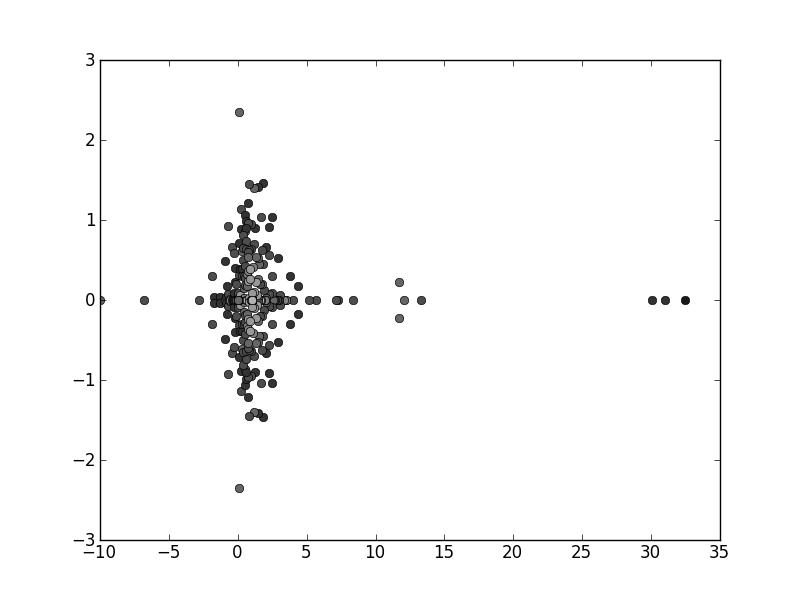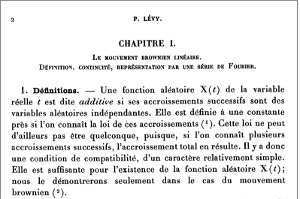# Month: December 2011

The beginning of winter is often the occasion to write programs. This year I wrote just for fun w2m.py, answering a question raised by my co-author Charles Bordenave. This Python 2.7 module+program consists in a spider which explores part of the World Wide Web, extracts the adjacency matrix, and computes its spectrum. Below the results for Wikipedia in Kabyle language. On the technical side, w2m.py has a simple object oriented code, which makes use of threading and numpy + matplotlib. I will hopefully convert it to Python 3.x some day (2to3). For Debian/GNU Linux, you may use apt-get install python-matplotlib python-scipy python-argparse in order to install all the necessary packages.

Related: The University of Florida Sparse Matrix Collection (pointed out by Charles Bordenave).Adjacency matrix spectrum for Wikipedia in Kabyle language (obtained in December 2011). The darkness of each point corresponds to the delocalization of the eigenvector.

Here is a chunk of the console output of w2m:

INFO:MainThread:_time_stamp():w2m.py 2011-12-28 12:27 http://kab.wikipedia.org/wiki
...
...
INFO:MainThread:show():number of http get errors: 30
INFO:MainThread:_time_stamp():w2m.py 2011-12-28 12:30 http://kab.wikipedia.org/wiki… Imaginons maintenant un mathématicien du genre de Paul Lévy, qui écrirait comme les mathématiciens parlent entre eux lorsqu’il n’y a pas d’étudiants dans la pièce, et lancerait quantité d’idées nouvelles : je pense que ses travaux seraient refusés par la plupart des grands journaux mathématiques. C’est un peu inquiétant pour notre avenir. ” in L’originalité de P.  Lévy en mathématiques, Paul-André Meyer, La Jaune et la Rouge, novembre 1973. Read more on-line.The Hoffman-Wielandt inequality is one of my favorite spectral inequalities in linear algebra. Before stating it, let us recall some basic concepts and objects.

Normal matrices. A matrix ${A\in\mathcal{M}_{n,n}(\mathbb{C})}$ is normal when ${AA^*=A^*A}$, where ${A^*}$ is its conjugate-transpose. This is equivalent to say that there exists a unitary matrix ${U}$ such that ${UAU^*}$ is diagonal (and the diagonal elements are precisely the eigenvalues of ${A}$). Every Hermitian and every unitary matrix is normal. A nilpotent matrix is never normal.

Trace norm. The trace norm on ${\mathcal{M}_{n,n}(\mathbb{C})}$ is defined for every ${A\in\mathcal{M}_{n,n}(\mathbb{C})}$ by

$\left\Vert A\right\Vert^2 :=\mathrm{Tr}(AA^*) =\mathrm{Tr}(A^*A) =\sum_{1\leq i,j\leq n} |A_{i,j}|^2.$

This is also known as the Frobenius, Schur, or Hilbert-Schmidt norm. As for the operator norm ${A\mapsto\left\Vert A\right\Vert_{2\rightarrow2}=\max_{\left\Vert x\right\Vert=1}\left\Vert Ax\right\Vert}$, the trace norm is unitary invariant, namely ${\left\Vert UAV\right\Vert=\left\Vert A\right\Vert}$ for any ${n\times n}$ unitary matrices ${U}$ and ${V}$ and any ${A\in\mathcal{M}_{n,n}(\mathbb{C})}$. The advantage of the trace norm over the operator norm lies in its convenient expression in terms of the matrix entries. Moreover, this norm is Hilbertian for the Hermitian product ${\left<A,B\right>=\mathrm{Tr}(AB^*)}$.

Hoffman-Wielandt inequality. It states that if ${A,B\in\mathcal{M}_{n,n}(\mathbb{C})}$ are normal, with respective eigenvalues ${\lambda_1(A),\ldots,\lambda_n(A)}$ and ${\lambda_1(B),\ldots,\lambda_n(B)}$, then, denoting by ${\mathcal{S}_n}$ the permutation group of ${\{1,\ldots,n\}}$,

$\min_{\sigma\in\mathcal{S}_n}\sum_{i=1}^n\left|\lambda_i(A)-\lambda_{\sigma(i)}(B)\right|^2 \leq\left\Vert A-B\right\Vert^2.$

In other words, the optimal matching of the spectra of ${A}$ and ${B}$ beats the trace norm. Let us give the original proof of Hoffman and Wielandt (1953). We have

$C:=UAU^*=\mathrm{diag}(\lambda_1(A),\ldots,\lambda_n(A))$

and

$D:=VBV^*=\mathrm{diag}(\lambda_1(B),\ldots,\lambda_n(B))$

for some ${n\times n}$ unitary matrices ${U}$ and ${V}$. By unitary invariance, we have

$\left\Vert A-B\right\Vert^2 =\left\Vert U^*CU-V^*DV\right\Vert^2 =\left\Vert CUV^*-UV^*D\right\Vert^2 =\left\Vert CW-WD\right\Vert^2$

where ${W:=UV^*}$. This gives, denoting ${P:=(|W_{i,j}|^2)_{1\leq i,j\leq n}}$,

$\left\Vert A-B\right\Vert^2 =\sum_{i,j=1}^n\left|(CW)_{i,j}-(WD)_{i,j}\right|^2 =\sum_{i,j=1}^nP_{i,j}\left|\lambda_i(A)-\lambda_j(B)\right|^2.$

The expression above is linear in ${P}$. Moreover, since ${W}$ is unitary, the matrix ${P}$ is doubly stochastic. If ${\mathcal{P}}$ is the set of ${n\times n}$ doubly stochastic matrices then

$\left\Vert A-B\right\Vert^2 \geq\inf_{Q\in\mathcal{P}}\Phi(Q) \quad\text{where}\quad \Phi(Q):=\sum_{i,j=1}^nQ_{i,j}\left|\lambda_i(A)-\lambda_j(B)\right|^2.$

But ${\Phi}$ is linear and ${\mathcal{P}}$ is convex and compact, and thus the infimum above is achieved for some extremal point ${Q}$ of ${\mathcal{P}}$. Now a celebrated theorem due to Birkhoff and von Neumann states that the extremal points of ${\mathcal{P}}$ are exactly the permutation matrices. Recall that ${P\in\mathcal{P}}$ is a permutation matrix when for a permutation ${\sigma\in\mathcal{S}_n}$ where ${\mathcal{S}_n}$ is the symmetric group, we have ${P_{i,j}=\delta_{\sigma(i),j}}$ for every ${i,j\in\{1,\ldots,n\}}$. This gives the final step of the proof:

$\left\Vert A-B\right\Vert^2 \geq \min_{\sigma\in\mathcal{S}_n}\sum_{i=1}^n\left|\lambda_i(A)-\lambda_{\sigma(i)}(B)\right|^2.$

The same proof provides without much efforts an upper bound, namely

$\max_{\sigma\in\mathcal{S}_n}\sum_{i=1}^n\left|\lambda_i(A)-\lambda_{\sigma(i)}(B)\right|^2 \geq \left\Vert A-B\right\Vert^2 \geq \min_{\sigma\in\mathcal{S}_n}\sum_{i=1}^n\left|\lambda_i(A)-\lambda_{\sigma(i)}(B)\right|^2.$

Coupling distance. Recall that the coupling distance between two probability measures ${\eta_1,\eta_2}$ on ${\mathbb{C}}$ with finite second moment is defined by

$d(\eta_1,\eta_2):=\inf\sqrt{\mathbb{E}(|X_1-X_2|^2)}$

where the infimum runs over the set of couple of random variables ${(X_1,X_2)}$ on ${\mathbb{C}\times\mathbb{C}}$ with ${X_1\sim\eta_1}$ and ${X_2\sim\eta_2}$. In the case where ${\eta_1=\frac{1}{n}\sum_{i=1}^n\delta_{a_i}}$ and ${\eta_2=\frac{1}{n}\sum_{i=1}^n\delta_{b_i}}$ where ${(a_i)_{1\leq i\leq n}}$ and ${(b_i)_{1\leq i\leq n}}$ are sequences in ${\mathbb{C}}$, we have, using the Birkhoff and von Neuman theorem on doubly stochastic matrices,

$d(\eta_1,\eta_2)^2= \frac{1}{n}\min_{\sigma\in\mathcal{S}_n}\sum_{i=1}^n\left|a_i-b_{\sigma(i)}\right|^2.$

This allows to rewrite the Hoffman-Wielandt inequality as

$nd^2(\mu_A,\mu_B)\leq \left\Vert A-B\right\Vert^2$

where

$\mu_A:=\frac{1}{n}\sum_{i=1}^n\delta_{\lambda_i(A)} \quad\text{and}\quad \mu_B:=\frac{1}{n}\sum_{i=1}^n\delta_{\lambda_i(B)}.$

Note that if ${A}$ and ${B}$ are Hermitian then ${(a_i)_{1\leq i\leq n}}$ and ${(b_i)_{1\leq i\leq n}}$ are in ${\mathbb{R}}$ and

$\min_{\sigma\in\mathcal{S}_n}\sum_{i=1}^n(a_i-b_{\sigma(i)})^2 =\sum_{i=1}^n(a_{(i)}-b_{(i)})^2.$

where ${a_{(1)}\leq\cdots\leq a_{(n)}}$ and ${b_{(1)}\leq\cdots\leq b_{(n)}}$ are the monotone reordering of both sequences. To see it, we may assume that the first sequence is already ordered as ${a_1\leq\cdots\leq a_n}$, and we observe that if ${b_i>b_{i+1}}$ for some ${i}$, then

$\begin{array}{rcl} (a_i-b_i)^2+(a_{i+1}-b_{i+1})^2 &=&(a_i-b_{i+1})^2+(a_{i+1}-b_i)^2+2(b_i-b_{i+1})(a_{i+1}-a_i)\\ &\geq& (a_i-b_{i+1})^2+(a_{i+1}-b_i)^2, \end{array}$

which allows to reorder the second sequence by using successive transpositions.

Singular values. The Hoffman-Wielandt inequality is also available for singular values of rectangular matrices. Recall that the singular values decomposition (SVD) of a rectangular matrix ${A\in\mathcal{M}_{m,n}(\mathbb{C})}$ states that there exists a couple of unitary matrices ${U}$ and ${V}$ of sizes ${m\times m}$ and ${n\times n}$ such that the ${m\times n}$ matrix ${D=U^*AV}$ is diagonal with ${D_{11}\geq\cdots\geq D_{rr}\geq0}$ where ${r=\min(m,n)}$ and ${D_{ii}=0}$ if ${i>r}$. The non-negative real numbers ${D_{11},\ldots,D_{rr}}$ are the singular values of ${A}$, denoted ${s_1(A),\ldots,s_r(A)}$. The largest singular value is nothing else but the operator norm ${s_1(A)=\max_{\left\Vert x\right\Vert=1}\left\Vert Ax\right\Vert =\left\Vert A\right\Vert_{2\rightarrow2}}$. Note that ${\left\Vert A\right\Vert^2=\mathrm{Tr}(AA^*)=s_1^2(A)+\cdots+s_r^2(A)}$. The matrices ${\sqrt{AA^*}}$ and ${\sqrt{A^*A}}$ have same spectrum ${s_1(A),\ldots,s_r(A)}$ up to multiplicity of ${0}$. There is also a nice linearization: the eigenvalues of the ${(m+n)\times(m+n)}$ Hermitian matrix

$\widetilde{A}=\begin{pmatrix}0&A\\A^*&0\end{pmatrix}$

are given by ${-s_1(A),\ldots,-s_r(A),0,\ldots,0,s_r(A),\ldots,s_1(A)}$ where ${0,\ldots,0}$ is a sequence of ${m+n-2r=\max(m,n)-\min(m,n)}$ zeros. The matrices ${\sqrt{AA^*}}$ and ${\widetilde{A}}$ are Hermitizations of the singular values decomposition.

We are now ready to state the Hoffman-Wielandt inequality for singular values of rectangular matrices. If ${A,B\in\mathcal{M}_{m,n}(\mathbb{C})}$ with respective singular values ${s_1(A)\geq\cdots\geq s_r(A)}$ and ${s_1(B)\geq\ldots\geq s_r(B)}$ then, setting ${r=\min(m,n)}$,

$\sum_{i=1}^r(s_i(A)-s_i(B))^2 =\min_{\sigma\in\mathcal{S}_r}\sum_{i=1}^r(s_i(A)-s_{\sigma(i)}(B))^2 \leq\left\Vert A-B\right\Vert^2.$

This follows immediately from the Hoffman-Wielandt inequality for the ${(m+n)\times(m+n)}$ Hermitian matrices ${\widetilde A}$ and ${\widetilde B}$ obtaiend by the linear Hermitization introduced above.

Non-normal matrices. As noticed by Hoffman and Wielandt, the inequality may not hold for non-normal matrices. Namely, take for instance

$A= \begin{pmatrix} 0 & 1 & 0 & \cdots & 0 \\ 0 & 0 & 1 & \cdots & 0 \\ \vdots & \vdots & \vdots & & \vdots \\ 0 & 0 & 0 & \cdots & 1 \\ 0 & 0 & 0 & \cdots & 0 \end{pmatrix} \quad\text{and}\quad B= \begin{pmatrix} 0 & 1 & 0 & \cdots & 0 \\ 0 & 0 & 1 & \cdots & 0 \\ \vdots & \vdots & \vdots & & \vdots \\ 0 & 0 & 0 & \cdots & 1 \\ \varepsilon_n & 0 & 0 & \cdots & 0 \end{pmatrix}$

in ${\mathcal{M}_n(\mathbb{R})}$, where say ${\varepsilon=1/n}$. The matrix ${A}$ is nilpotent, and ${A-B}$ has rank one and small norm. We have ${\left\Vert A-B\right\Vert^2=\varepsilon_n^2\rightarrow0}$. On the other hand ${\lambda_1(A)=\cdots=\lambda_n(A)=0}$, while ${B^n=\varepsilon_nI_n}$ which gives ${\lambda_k(B)=\varepsilon_n^{1/n}\mathrm{e}^{2i\pi k/n}}$ for all ${1\leq k\leq n}$, and therefore, for any ${\sigma\in\mathcal{S}_n}$, ${\sum_{k=1}^n|\lambda_k(A)-\lambda_{\sigma(k)}(B)|^2 =n\varepsilon_n^{2/n}=n\mathrm{e}^{2\log(n)/n}\rightarrow\infty}$, hence the contradiction. Hoffman and Wielandt gave the ${2\times 2}$ counter example

$A= \begin{pmatrix} 0 & 0 \\ 0 & 4 \end{pmatrix} \quad\text{and}\quad B= \begin{pmatrix} -1 & -1 \\ +1 & +1 \end{pmatrix},$

${A}$ is normal, ${B}$ is not, ${\left\Vert A-B\right\Vert^2=12}$, ${\sum_{k=1}^2(\lambda_k(A)-\lambda_{\sigma(k)}(B))^2=16}$ for any ${\sigma\in\mathcal{S}_n}$.

Infinite dimensions. The Hoffman-Wielandt inequality remains valid for infinite dimensional Hermitian or Hilbert-Schmidt operators, provided that the sequence of eigenvalues are defined in a suitable way. The proof can be based by reduction on the finite dimensional case. We refer to the work of Bhatia and Elsner cited below for details and references.

${p}$-norms. Set ${p\geq1}$. The Hoffman-Wielandt inequality for Hermitian matrices can be extended to ${p}$-norms. More precisely it can be shown that for any Hermitian matrices ${A,B\in\mathcal{M}_{n,n}(\mathbb{C})}$ with respective eigenvalues ${\lambda_1(A)\geq\cdots\geq\lambda_n(A)}$ and ${\lambda_1(B)\geq\cdots\geq\lambda_n(B)}$, we have

$\sum_{i=1}^n(\lambda_i(A)-\lambda_{i}(B))^p \leq \left\Vert A-B\right\Vert_p^p$

where ${\left\Vert\cdot\right\Vert_p=(s_1^p(\cdot)+\cdots+s_n(\cdot)^p)^{1/p}}$ is the Schatten ${p}$-norm. This follows from a generalization by Lidskii and Wielandt of the Courant-Fischer minimax variational formulas to cumulative sums of ordered eigenvalues of Hermitian matrices, related to the majorization concept. The extension beyond Hermicity to normal matrices is problematic, and we refer to the work of Bhatia and Elsner cited below for details and references.

Related inequalities. There are plenty of inequalities on the variation of the spectrum by perturbation. For instance the Weyl interlacing inequality states that if ${A,B\in\mathcal{M}_{n,n}(\mathbb{C})}$ are Hermitian with respective eigenvalues ${\lambda_1(A)\geq\cdots\geq\lambda_n(A)}$ and ${\lambda_1(B)\geq\cdots\geq\lambda_n(B)}$ and if ${\lambda_1(A+B)\geq\cdots\geq\lambda_n(A+B)}$ are the eigenvalues of ${A+B}$ then ${\lambda_{i+j-1}(A+B)\leq\lambda_i(A)+\lambda_j(B)}$ for any ${1\leq i,j\leq n}$ with ${i+j-1\leq n}$. They follow from the Courant-Fischer minimax variational formulas for the eigenvalues of Hermitian matrices. From Weyl’s inequalities we get in particular that

$\max_{1\leq i\leq n}|\lambda_i(A)-\lambda_i(B)| \leq\left\Vert A-B\right\Vert_{2\rightarrow2}.$

In the same spirit, and beyond Hermitian matrices, the Bauer-Fike inequality states that if ${A\in\mathcal{M}_{n,n}(\mathbb{C})}$ is diagonalizable, say ${A=PDP^{-1}}$ with ${D}$ diagonal, and if ${B\in\mathcal{M}_{n,n}(\mathbb{C})}$, then, for any eigenvalue ${\mu}$ of ${B}$, there exists an eigenvalue ${\lambda}$ of ${A}$ such that

$|\lambda-\mu| \leq\kappa(P)\left\Vert A-B\right\Vert_{2\rightarrow2}$

where ${\kappa(P)=\left\Vert P\right\Vert_{2\rightarrow2}\left\Vert P^{-1}\right\Vert_{2\rightarrow2}=\frac{s_1(P)}{s_n(P)}}$ is the condition number of ${P}$. It follows from

$0=\det(B-\mu I_n)=\det(D-\mu I_n)\det((D-\mu I_n)^{-1}P^{-1}(B-A)P+I_n).$

Further reading. Here are some interesting references.

• Alan Hoffman and Helmut Wielandt. The variation of the spectrum of a normal matrix. Duke Math. J. 20, (1953). 37-39.
• Rajendra Bhatia and Ludwig Elsner. The Hoffman-Wielandt inequality in infinite dimensions. Proc. Indian Acad. Sci. Math. Sci. 104 (1994), no. 3, 483-494.
• Roger Horn, and Charles Johnson. Matrix analysis. Second edition. Cambridge University Press, Cambridge, 2013. xviii+643 pp. ISBN: 978-0-521-54823-6
• Roger Horn and Charles Johnson. Topics in matrix analysis. Corrected reprint of the 1991 original. Cambridge University Press, Cambridge, 1994. viii+607 pp. ISBN: 0-521-46713-6
• Rajendra Bhatia. Positive definite matrices.  paperback edition of the 2007 original. Princeton Series in Applied Mathematics. Princeton University Press, Princeton, NJ, 2007. ix+254 pp. ISBN: 978-0-691-16825-8; 978-0-400-82778-7
• Rajendra Bhatia. Matrix analysis. Graduate Texts in Mathematics, 169. Springer-Verlag, New York, 1997. xii+347 pp. ISBN: 0-387-94846-5
• Bhatia, Rajendra. Perturbation bounds for matrix eigenvalues. Reprint of the 1987 original. Classics in Applied Mathematics, 53. Society for Industrial and Applied Mathematics (SIAM), Philadelphia, PA, 2007. xvi+191 pp. ISBN: 978-0-898716-31-3
• Tosio Kato. Perturbation theory for linear operators. Reprint of the 1980 edition. Classics in Mathematics. Springer-Verlag, Berlin, 1995. xxii+619 pp. ISBN: 3-540-58661-X

Note. Alan Hoffman (1924 – ) is also famous for the Hoffman-Singleton graph.

Note. Helmut Wielandt (1910 – 2001) devoted his doctoral thesis and habilitation to permutation groups. He wrote a book on finite permutation groups. From 1941 he did research on meteorology, cryptology and aerodynamics. In 1942 he was attached to the Kaiser Wilhelm Institute and the Aerodynamics Research Institute at Göttingen. He said “… I had to work on vibration problems. I am indebted to that time for valuable discoveries: on the one hand the applicability of abstract tools to the solution of concrete problems, on the other hand, the – for a pure mathematician – unexpected difficulty and unaccustomed responsibility of numerical evaluation. It was a matter of estimating eigenvalues of non-self-adjoint differential equations and matrices.” Source: MacTutor.

Syntax · Style · Tracking & Privacy.